# Currency Market, Commodity Market, and Government Securities

## 5.1 - Futures Calendar spread

The futures contract should always trade at a premium than the spot, assuming all other things are equal. As we all know, this can be attributed the Futures pricing formula's interest rate factor (cost to carry). This was discussed in theFuture Module..Arbitrage opportunities can be created by any variation to this equation.

This scenario is where arbitrage opportunities exist between Spot futures.

Futures trading at a lower rateSuppose the spot price is 100 and the fair value for its future is 105. You can calculate the fair value of the future using the futures pricing method. The 'no arbitration spread' is the difference in spot and future's fair values, i.e. 105 - 100 = 5.

This means that if the future trades at 98 for any reason (market mispricing), there is a spread of 7 (105–98) between spot and future. This spread can be captured.

You can buy the future at 99 and then simultaneously sell the spot at 100. The spread will be captured once the futures and spot expire.

If you don't understand the above, I encourage you to review the Futures module chapter (link above).

If the futures trade at an even higher price than its fair value, one can also capture the spread by selling futures and purchasing the spot.

This is something we have already learned and it is very simple. Practical reasons, such as arbitrage trades involving spot or futures, cannot be made with the USDINR contract. The reason is that the USDINR spot markets are not accessible to retail.

How can one trade spreads in the currency segment. This is easy. Rather than spot-future spreads, one must identify the spread between two futures contracts that expire at different dates. This spread is also known by the 'Calendar Spread.

You decide whether the spread between two futures contracts is normal or abnormal by creating a calendar spread. The long-dated futures contract, regardless of its date, will always trade at an advantage over the shorter term futures contract. The August month futures contract will trade at a premium compared to the July month. A certain amount of spread is expected between the two contracts. There are situations when the spread is higher or lower than normal, and these are opportunities.

Today's USD INR July Futures are trading at 67.3075 and the August contract at 67.6900.

Spread is the difference between two futures contracts.

67.6900 to 67.3075

= 0.3825

Let's say that you believe this spread of 0.3825 is too high. It should be 0.2000, not 0.3825. This is an arbitrage opportunity, and you can make -

0.3825 - 1.2000

= 0.1825

You will need to simultaneously buy and sell Aug Futures in order to capture the spread.

Long July Futures at 67.32575

Short August Futures at 66.7900

A "trade" is a trade that has both a long current expiry and a shorter further term expiry.Future Bull Spread". A '.Futures Bear spread" is when you must shorten the current month's expiry and take a longer term for the next month.

However, once the "Future Bull Spread" is set up, you must monitor the trades and close the trade when the spread converges below 0.2000. Profitable trades are those that occur when the spread converges to 0.2000 or lower.

1. When the Aug (shorter) leg rises while the July (longer) leg falls
2. The long leg rises and the short leg stays the same.
3. The long leg raises and the short leg rises at a slower rate.
4. The shorter leg falls quicker than the longer leg
5. If the long leg is not changed, and the short leg falls,

Is the spread likely to converge? If so, when will it converge. Why should it converge Which of the above scenarios will actually work out? The answer depends on how well your spread knowledge is. Backtesting the spread will help you understand it better. Backtesting techniques are a subject for another day. However, I would like to show how simple it is to sell spreads from your trading terminal.

What would it look like if you could buy and sell the spread directly? In the example above, we concluded that 0.3825 was an excessively priced spread. To capture this spread, you must place two orders. Purchase July Futures and Sell August Futures

Executing these trades can be inefficient mainly because of execution risk. Prices could change between the time you buy/sell the contracts and the spread might not look as attractive.

This makes it very convenient to purchase the spreads directly, and not have to deal with two contracts. Zerodha customers have access to NEST traders, which allows them to trade the spreads directly. This will be made available in Pi and Kite in the future.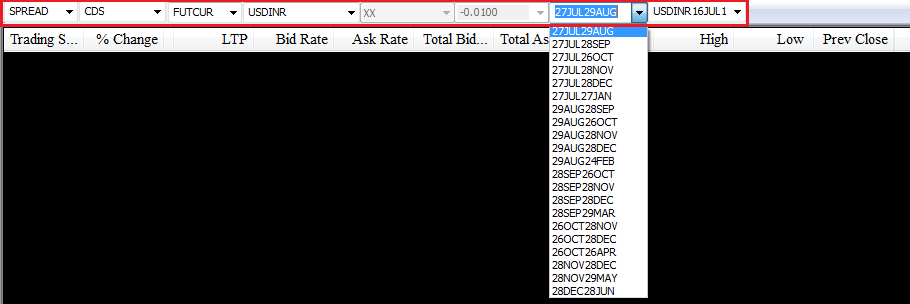As you may have guessed, the red part is the market watch. Start from the left

1. If you select 'Spread' from the dropdown, it means that we are interested spread contracts.
2. After choosing spreads, we select CDS from dropdown to indicate that currency derivatives are the segment.
3. FUTCUR means that we are interested in future contracts within CDS spreads.
4. USDINR means that we are interested USDINR contracts.
5. You can see the full dropdown menu here. As you can see there are many spreads. We are only interested in July-August spreads, so we have chosen that one.

After we have set up the above-market watch we submit this to load it. Here is what it looks like: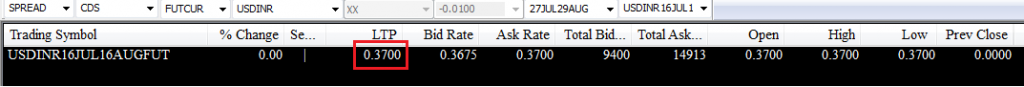Below is the spread's most recent traded price. This spread instrument represents the spread between the July and August contracts, as you can see.

The spread should trade at 0.3825, not 0.3700. What makes you believe there is a price differential?

This will be explained from my understanding. I may be wrong so please comment! We are also a little off-topic, so please keep your eyes on the main topic. How to trade spreads.

Take a look at this snapshot -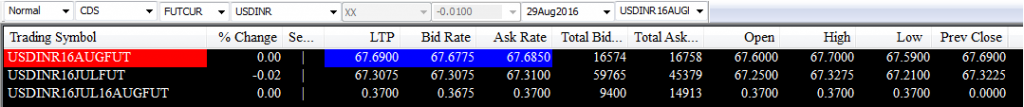Let's not talk about spread contracts. For now, let's say you want to create a Future Bull Spread (buy July and sell Aug) contract.

July Contract at Ask Rate - 67.3100

Sell Aug contract at the BID Rate - 66.77575

Spread = 67.6775- 67.3100 = 0.3675

If you wanted to create a Future Bear Spread, you would essentially:

August Contract at Ask Rate - 66.75050

Sell July contract at Bid rate - 67.3075

There are two possible spreads depending on what you plan to do. future bull/bear spread.

The question now is: Which spread price should it reflect? It could be the Future Bull Spread, or the Future Bear spread.

Spread trades are close to the average spread of both spreads. The market spread is actually 0.3700. In this instance, the average spread is 0.3725. Why is it 0.3700, and not 0.3725? This could be due to either a terminal not capturing the latest quote or a lack of liquidity.

Another explanation could be that spread is too expensive!

But, let's get back to the main point, i.e. Selling or buying the spread. After the spread instrument has been loaded, you can simply select the instrument from the market watch and hit F1 or F2 to buy and sell.

This is what you will see when you invoke the buy order window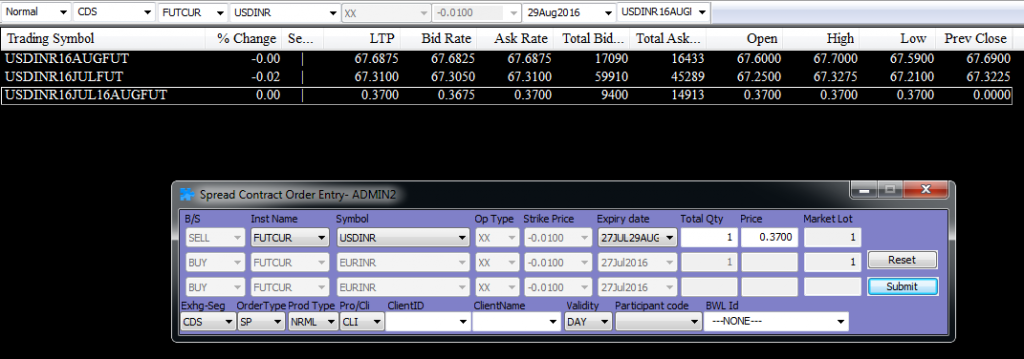The spread details are already pre-populated in the window. You may need to adjust the quantity to meet your lot size requirements. To place an order, press submit

It's as easy as that!

## Stats: USD INR 5.3

It would be interesting to examine some statistics about the USD-INR pair. I downloaded the USD-INR Spot data from RBI.

Let's start with the USD INR chart over the past 8 years (July 2008 to Jul 2016).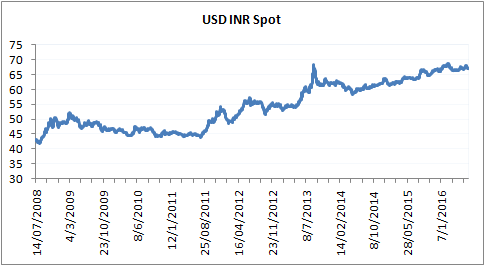The US Dollar has risen against the Indian Rupee in the past 8 years. This is quite logical, as our economy has virtually stagnated over the past years.

Take a look at this chart of USD INR's daily return.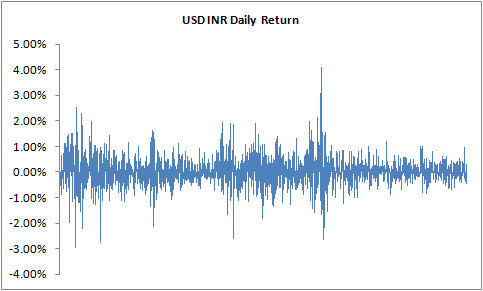These are some of the interesting parameters we can observe.

USD INR's average daily return is 0.025%. You will notice that USD INR has a lower volatility than Nifty 50, or any other indices. The volatility numbers further illustrate this fact.

• Daily Standard Deviation (last 8 years): 0.567%
• Daily standard deviation (2015) = 0.311%
• Annualized standard deviation (2015) - 4.94%

These numbers clearly are lower than the Nifty 50’s annualized volatility (0.82%) and daily volatility (15.71%), respectively.

Additionally, I ran a correlation function for Nifty 50 and USDINR. Before I tell you the answer, I want to ask you to guess what this correlation would look like.

Here's a quick explanation for those who don’t know what correlation means.

The correlation between two variables is a way to see how they interact with each other. Correlation can be described as a number that varies from -1 to +1. If the correlation between two variables is +0.75, it means that there are two things.

1. The number is positive correlated if it has a plus before it. They move in the same direction.
2. This number is indicative of the strength and power of this movement. The tendency of the variables to move in tandem is higher if they are closer to +1 or -1.
3. A correlation of 0 indicates that the variables are not related.

The above shows that the correlation of +0.75 indicates that the variables not only move in the same direction, but also tends to move closely together. The correlation does not indicate the magnitude of the move. All that suggests is that it is likely that the two variables will move in the same direction. If Stock A moves by 3% and the correlation between stock B and stock A is +0.75, it doesn't mean Stock B will also move 3%. The correlation only suggests that Stock B will rise in the same direction as Stock A.

There is a twist to this story. Let's say stock A and stock B are correlated at 0.75 and their daily average returns of Stock A is 0.9% and Stock B is 1.2%. On any given day, Stock A will move above its daily average return 0.9% and stock B will likely move higher than its daily return of 1.2%.

A correlation of -0.75 means that the variables move in opposite directions (indicated with the -ve sign). Let's say stock A goes up by 2.5%. If there is a correlation, it means that Stock B is most likely to fall, but how much is not known.

One more thing about correlation while we're at it. This is for people who are interested in correlation math. Only if the data series are'stationary around their mean', the correlation data will make sense. What does this mean? It simply means that the average values should be maintained in the data set. You can also take a look at the graph showing the daily returns for USD INR. We have republished the graph for your convenience.This is the daily average return. It is 0.025%. The daily average return is 0.025%. This means that even though the returns go up or down, they eventually stick to the average value. This property is known as "stationary around the mean" in data-series.Stock or commodity or currency returns are invariably stationary, but the Stock or commodity or currency prices are not stationary as they tend to trend.

Are you confused? Confused?

It takes only 2 steps to calculate the correlation between two variables.

4. Calculate the daily returns
5. Excel has the function '=Correl'.
6. Enter to get the correlation between these variables.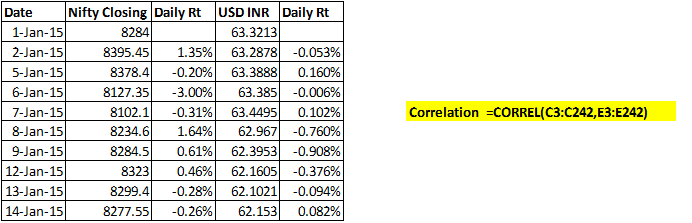Keep in mind that the correlation between Stock A and Stock B is equal to the correlation between Stock A and Stock A.

You should have a good understanding of correlation. I'm going to ask you again.

What would be the correlation between USDINR 50 and Nifty 50? You can guess the correlation between USDINR and Nifty 50, but not the actual number.

Let's try to deduce: If the markets, as a whole economy, are performing well, then foreign investors will tend to invest in the markets. This is a sign that dollars are flowing into the country. Dollars are sold in order to convert to Rupee. This basically means that dollars are sold to get converted into Rupees. Naturally, this will increase Rupee strength. The USDINR falls while the Nifty50 rises. You can also apply the same logic if you look at it in reverse, i.e. USDINR rising, while the market falls.

This would mean that USDINR and Nifty 50 should be invertedly related. This is actually true. The correlation value for 2015 data is -0.12267.